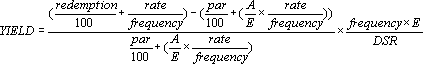## Excel Office

Excel How Tos, Tutorials, Tips & Tricks, Shortcuts

# YIELD function: Description, Usage, Syntax, Examples and Explanation

## What is YIELD function in Excel?

YIELD function is one of Financial functions in Microsoft Excel that returns the yield on a security that pays periodic interest. Use YIELD to calculate bond yield.

## Syntax of YIELD function

YIELD(settlement, maturity, rate, pr, redemption, frequency, [basis])

Important: Dates should be entered by using the DATE function, or as results of other formulas or functions. For example, use DATE(2008,5,23) for the 23rd day of May, 2008. Problems can occur if dates are entered as text.

The YIELD function syntax has the following arguments:

• Settlement: The security’s settlement date. The security settlement date is the date after the issue date when the security is traded to the buyer.
• Maturity: The security’s maturity date. The maturity date is the date when the security expires.
• Rate: The security’s annual coupon rate.
• Pr: The security’s price per \$100 face value.
• Redemption: The security’s redemption value per \$100 face value.
• Frequency: The number of coupon payments per year. For annual payments, frequency = 1; for semiannual, frequency = 2; for quarterly, frequency = 4.
• Basis(Optional): The type of day count basis to use.
 Basis Day count basis 0 or omitted US (NASD) 30/360 1 Actual/actual 2 Actual/360 3 Actual/365 4 European 30/360

## YIELD formula explanation

• Microsoft Excel stores dates as sequential serial numbers so they can be used in calculations. By default, January 1, 1900 is serial number 1, and January 1, 2008 is serial number 39448 because it is 39,448 days after January 1, 1900.
• The settlement date is the date a buyer purchases a coupon, such as a bond. The maturity date is the date when a coupon expires. For example, suppose a 30-year bond is issued on January 1, 2008, and is purchased by a buyer six months later. The issue date would be January 1, 2008, the settlement date would be July 1, 2008, and the maturity date would be January 1, 2038, which is 30 years after the January 1, 2008, issue date.
• Settlement, maturity, frequency, and basis are truncated to integers.
• If settlement or maturity is not a valid date, YIELD returns the #VALUE! error value.
• If rate < 0, YIELD returns the #NUM! error value.
• If pr ≤ 0 or if redemption ≤ 0, YIELD returns the #NUM! error value.
• If frequency is any number other than 1, 2, or 4, YIELD returns the #NUM! error value.
• If basis < 0 or if basis > 4, YIELD returns the #NUM! error value.
• If settlement ≥ maturity, YIELD returns the #NUM! error value.
• If there is one coupon period or less until redemption, YIELD is calculated as follows:where:
• A = number of days from the beginning of the coupon period to the settlement date (accrued days).
• DSR = number of days from the settlement date to the redemption date.
• E = number of days in the coupon period.
• If there is more than one coupon period until redemption, YIELD is calculated through a hundred iterations. The resolution uses the Newton method, based on the formula used for the function PRICE. The yield is changed until the estimated price given the yield is close to price.

## Example of YIELD function

Steps to follow:

1. Open a new Excel worksheet.

2. Copy data in the following table below and paste it in cell A1

Note: For formulas to show results, select them, press F2 key on your keyboard and then press Enter.

You can adjust the column widths to see all the data, if need be.

 Data Description 15-Feb-08 Settlement date 15-Nov-16 Maturity date 5.75% Percent coupon 95.04287 Price \$100 Redemption value 2 Frequency is semiannual (see above) 0 30/360 basis (see above) Formula Description (Result) Result =YIELD(A2,A3,A4,A5,A6,A7,A8) The yield, for the bond with the terms above (0.065 or 6.5%) 6.5%